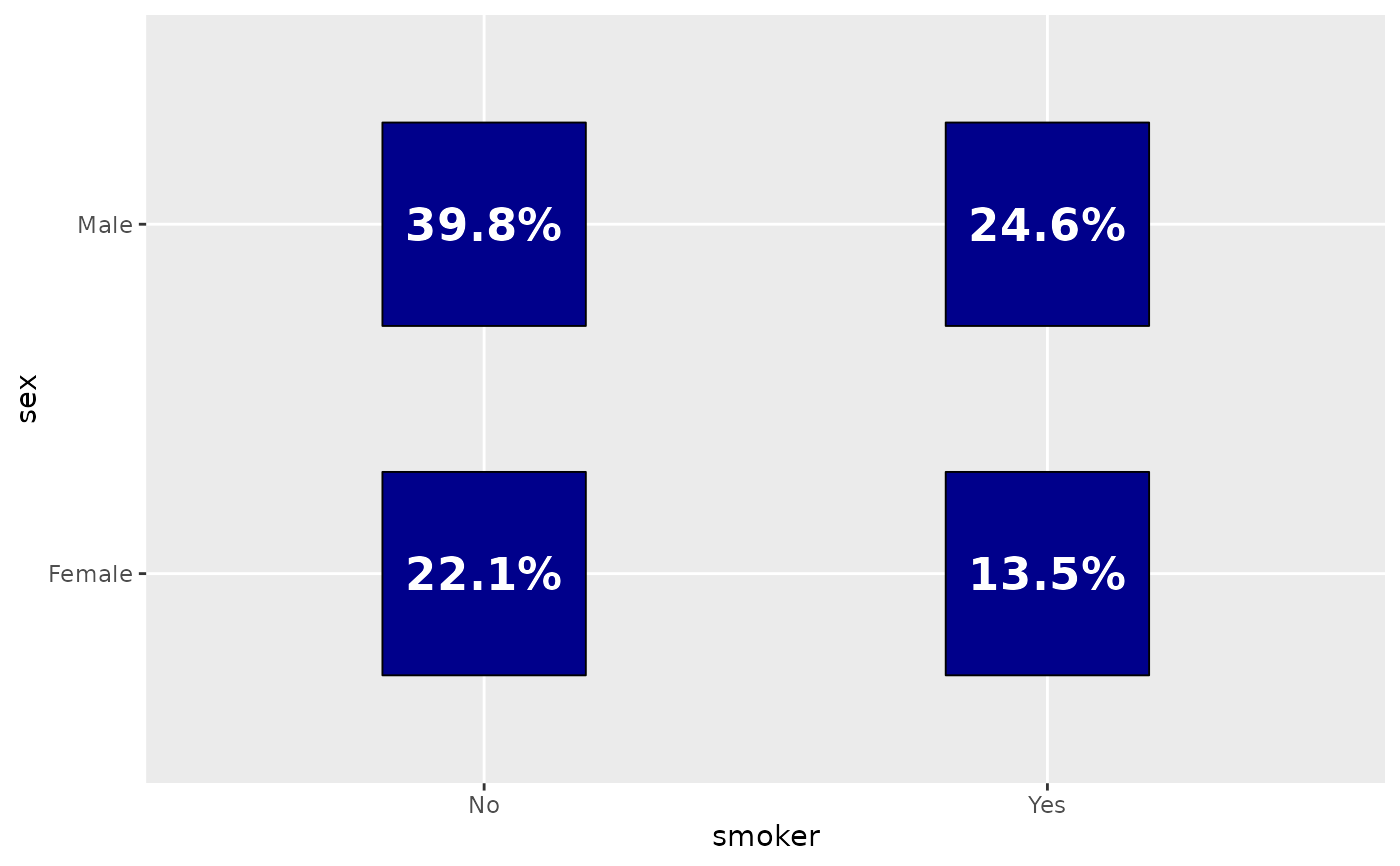Plot the number of observations by using square points with proportional areas. Could be filled according to chi-squared statistics computed by stat_cross(). Labels could also be added (see examples).

ggally_cross(data, mapping, ..., scale_max_size = 20, geom_text_args = NULL)

## Arguments

data data set using aesthetics being used other arguments passed to ggplot2::geom_point() max_size argument supplied to ggplot2::scale_size_area() other arguments passed to ggplot2::geom_text()

## Author

Joseph Larmarange

## Examples

# Small function to display plots only if it's interactive
p_ <- GGally::print_if_interactive

data(tips, package = "reshape")
p_(ggally_cross(tips, mapping = aes(x = smoker, y = sex)))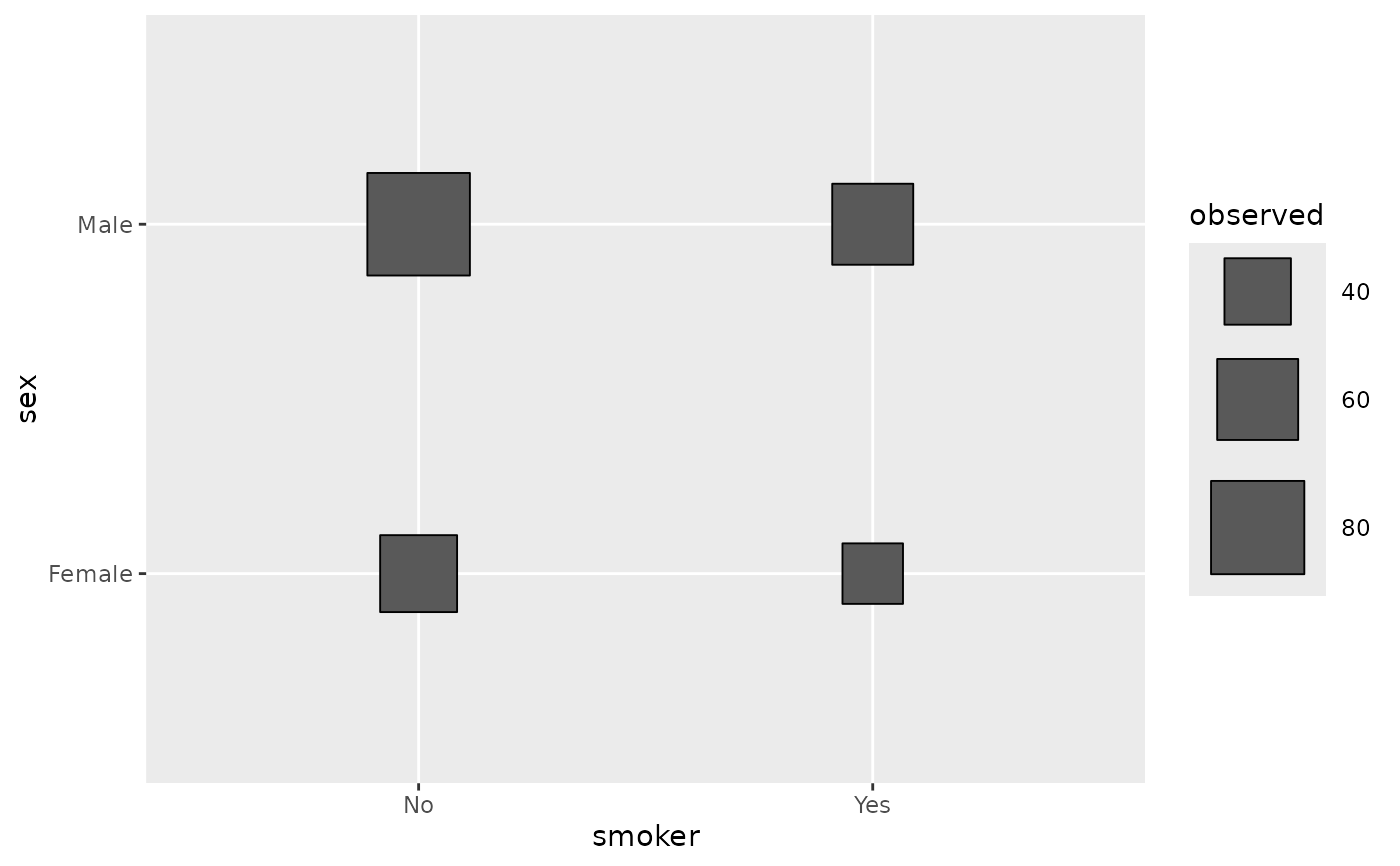p_(ggally_cross(tips, mapping = aes(x = day, y = time)))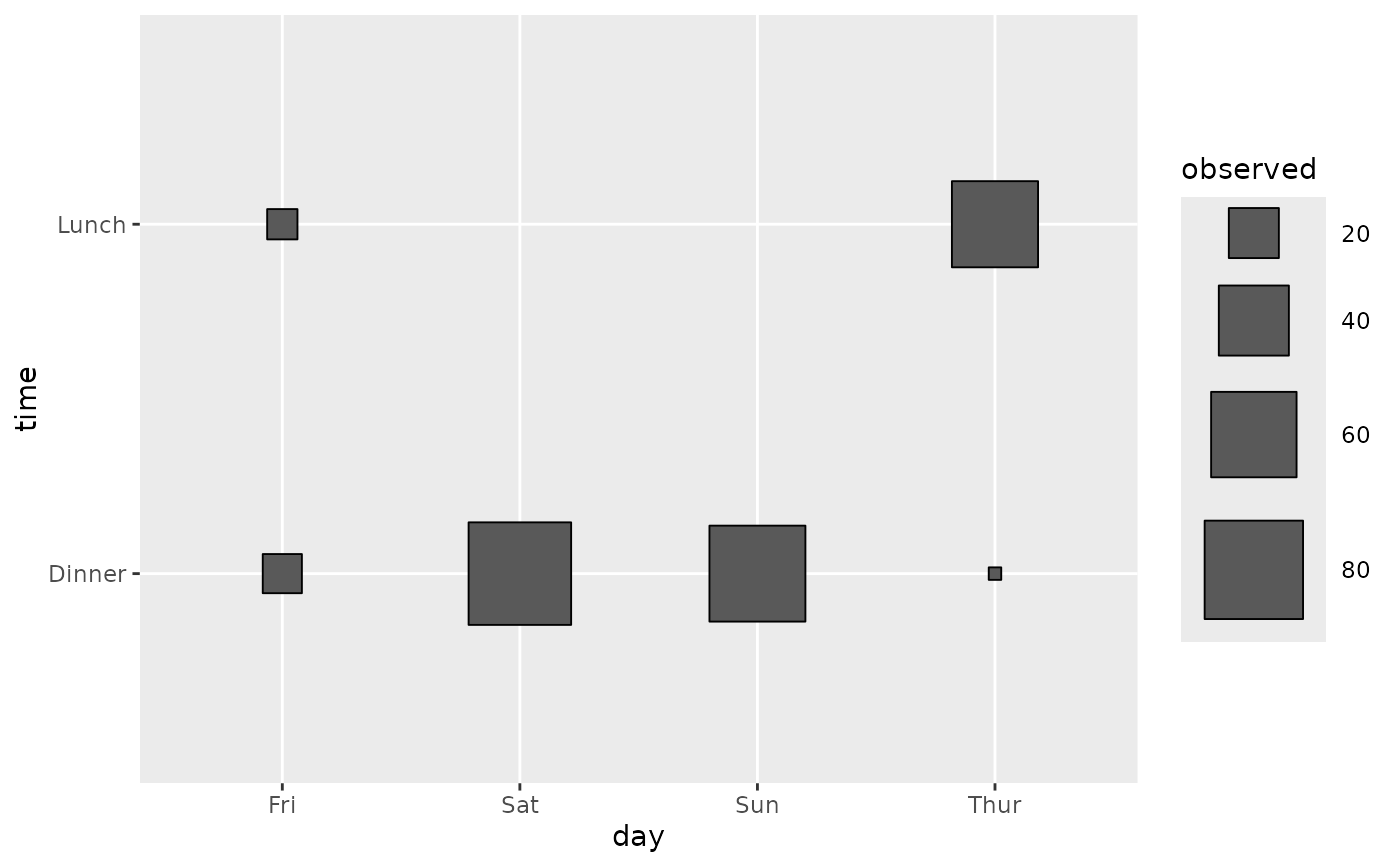# Custom max size
p_(ggally_cross(tips, mapping = aes(x = smoker, y = sex)) +
scale_size_area(max_size = 40))
#> Scale for 'size' is already present. Adding another scale for 'size', which
#> will replace the existing scale.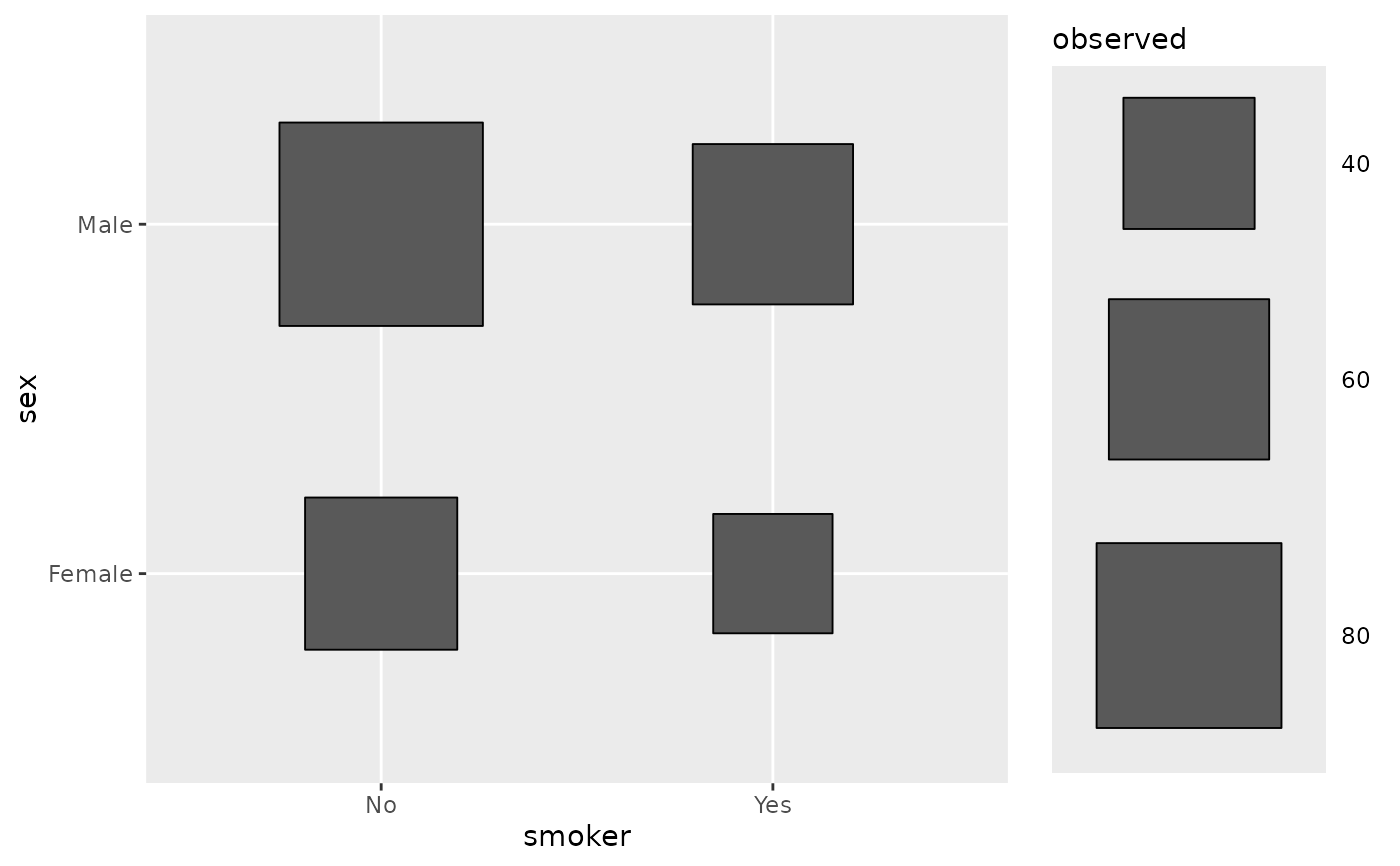# Custom fill
p_(ggally_cross(tips, mapping = aes(x = smoker, y = sex), fill = "red"))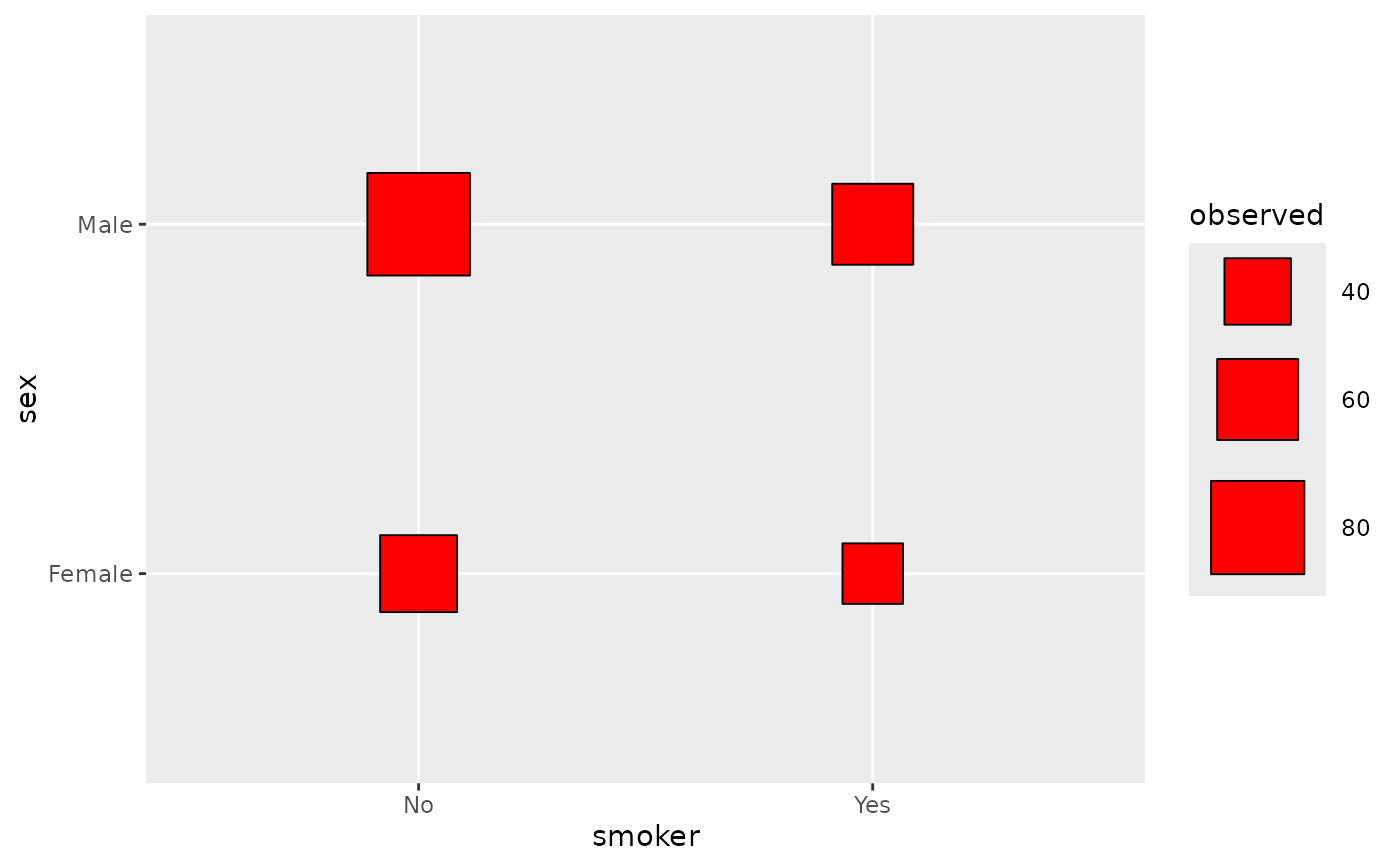# Custom shape
p_(ggally_cross(tips, mapping = aes(x = smoker, y = sex), shape = 21))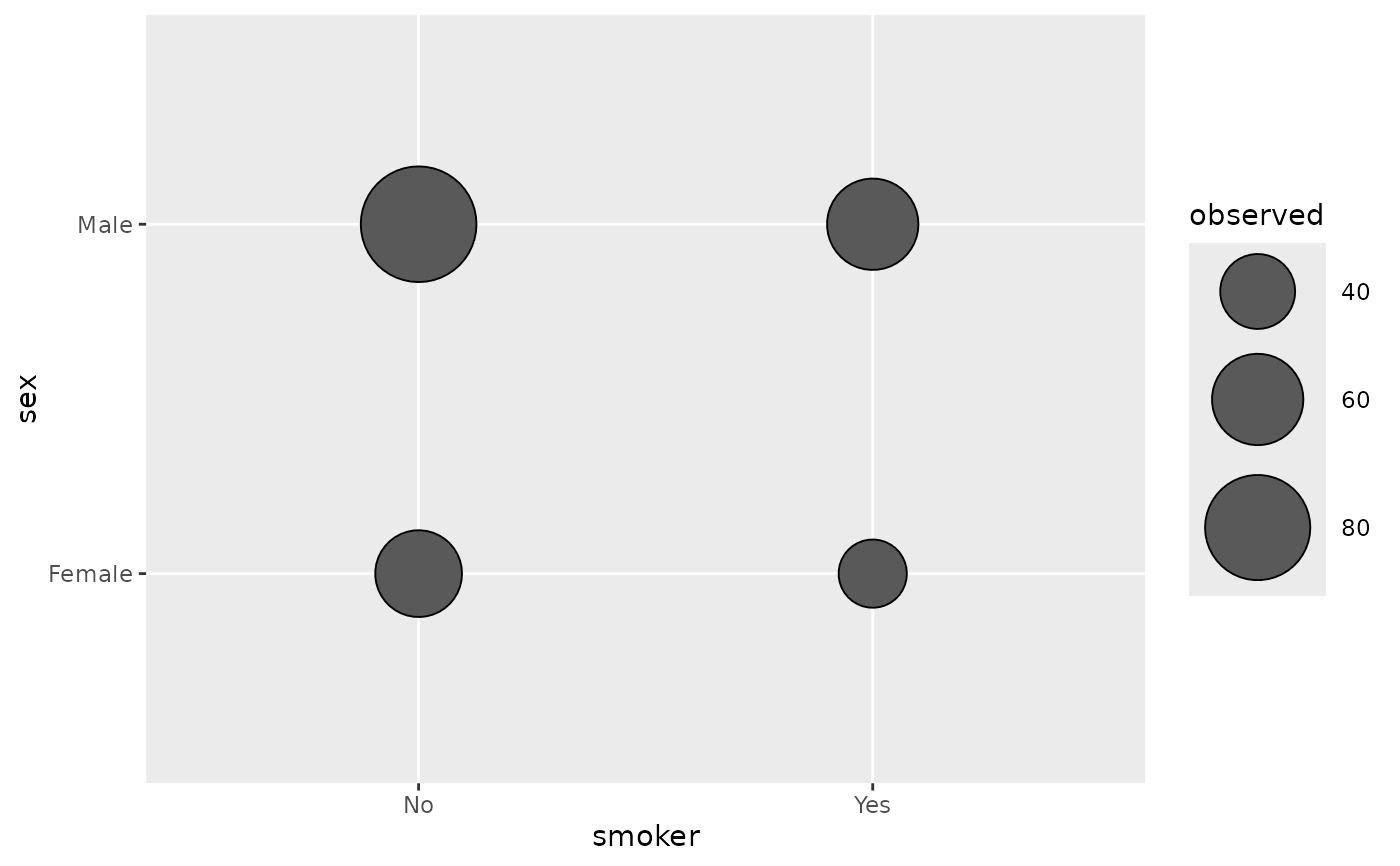# Fill squares according to standardized residuals
d <- as.data.frame(Titanic)
p_(ggally_cross(
d,
mapping = aes(x = Class, y = Survived, weight = Freq, fill = after_stat(std.resid))
) +
scale_fill_steps2(breaks = c(-3, -2, 2, 3), show.limits = TRUE))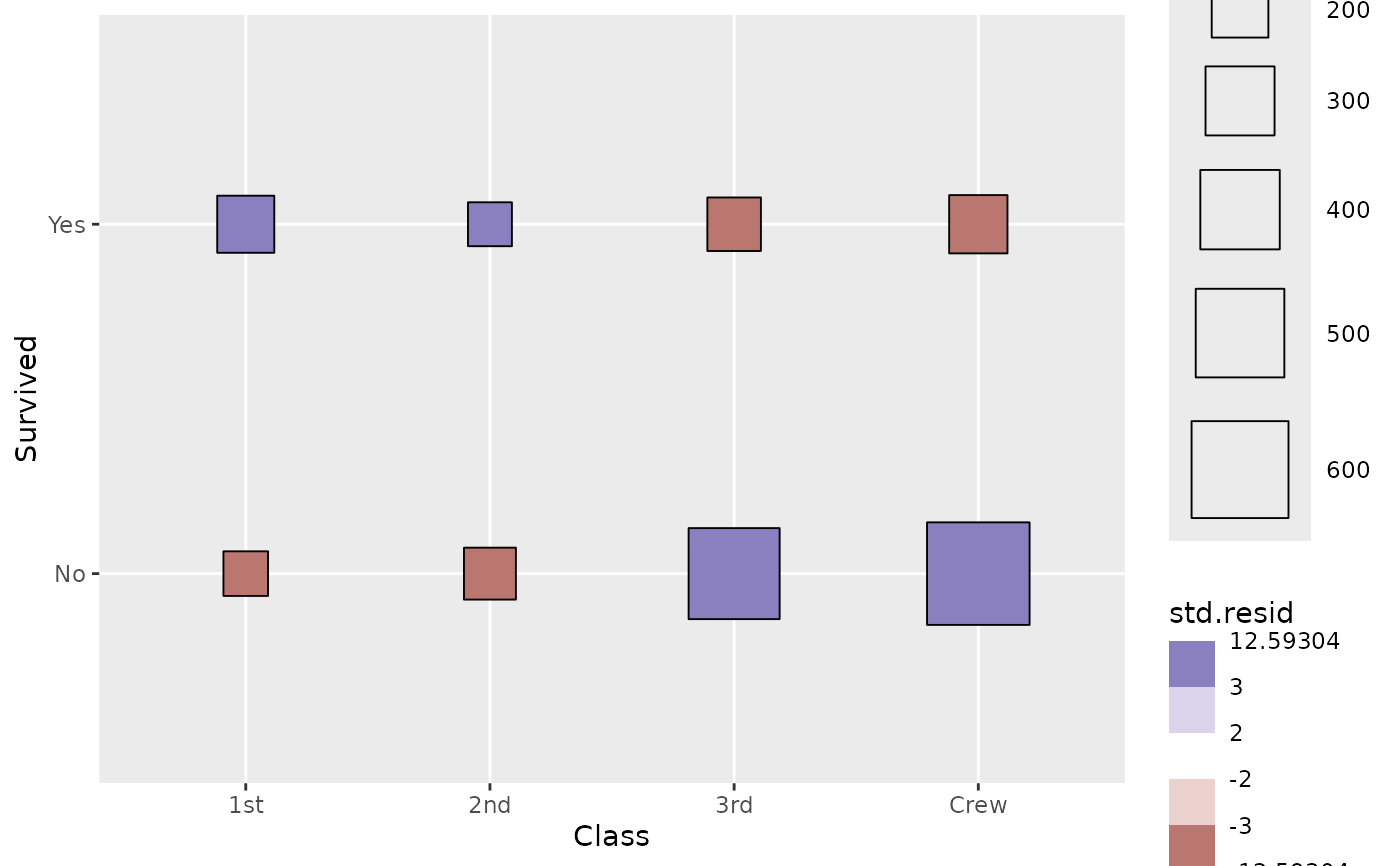p_(ggally_cross(
tips,
mapping = aes(
x = smoker, y = sex, colour = smoker,
label = scales::percent(after_stat(prop))
)
))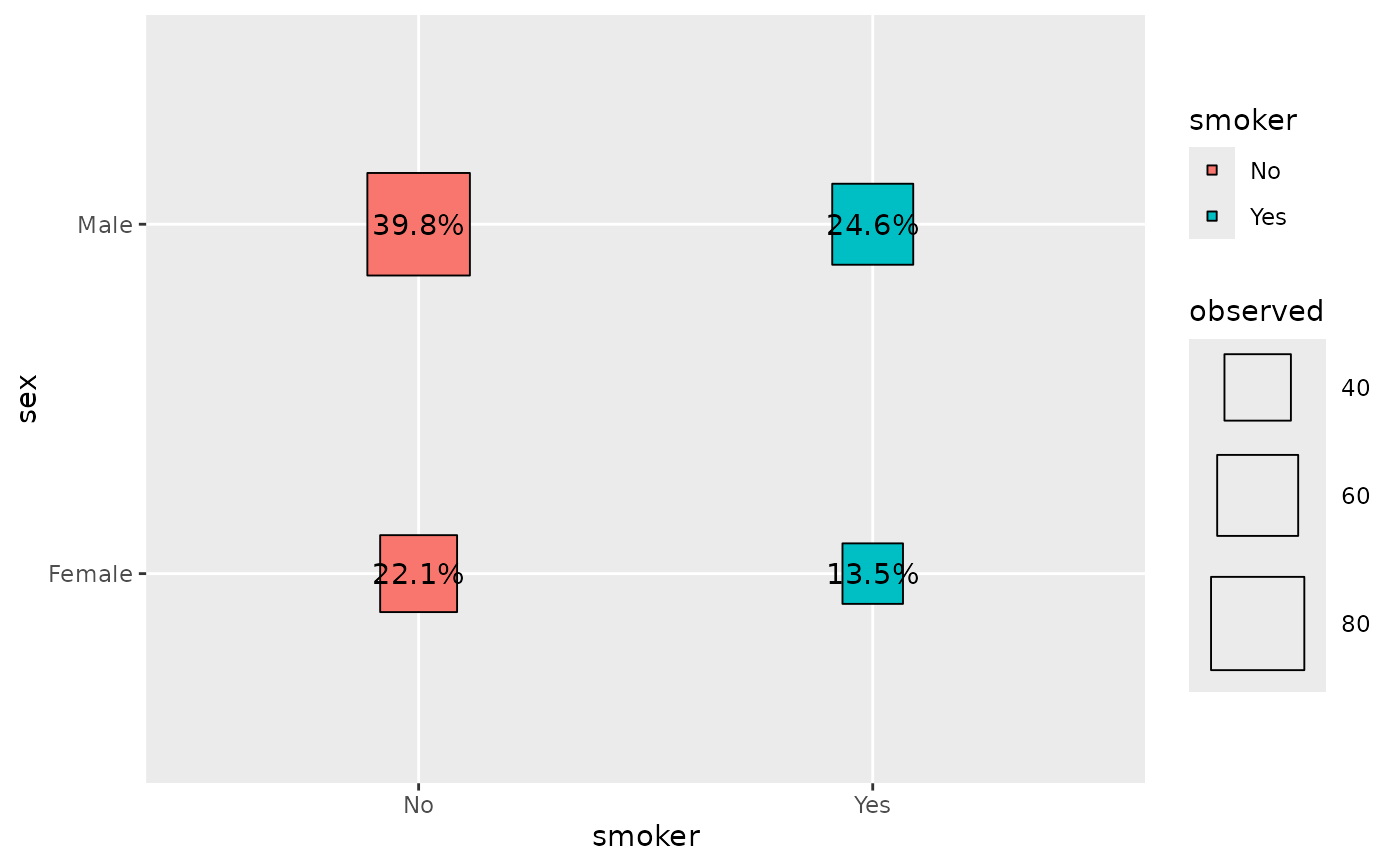# Customize labels' appearance and same size for all squares
p_(ggally_cross(
tips,
mapping = aes(
x = smoker, y = sex,
size = NULL, # do not map size to a variable
label = scales::percent(after_stat(prop))
),
size = 40, # fix value for points size
fill = "darkblue",
geom_text_args = list(colour = "white", fontface = "bold", size = 6)
))